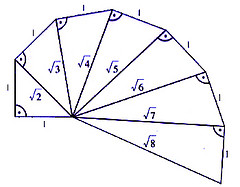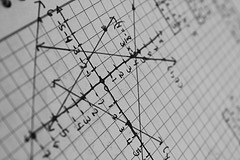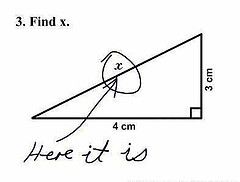FAIL (the browser should render some flash content, not this).Math 10 Principles Ch 1

Sequences and SeriesAt BCMath.ca we offer hundreds of free online math lessons for students and teachers. Help support our site by clicking on the button above to donate through Paypal.Math 10 Principles Ch 2Math 10 Principles Ch 3

Slopes, Midpoints, and Distance FormulasMath 10 Principles Ch 4

Graphing Linear EquationsMath 10 Principles Ch 5

FunctionsMath 10 Principles Ch 6

Factoring PolynomialsMath 10 Principles Ch 7

Simplifying Rational ExpressionsMath 10 Principles Ch 8

TrigonometryMath 10 Principles Ch 9

Statistics and Probability